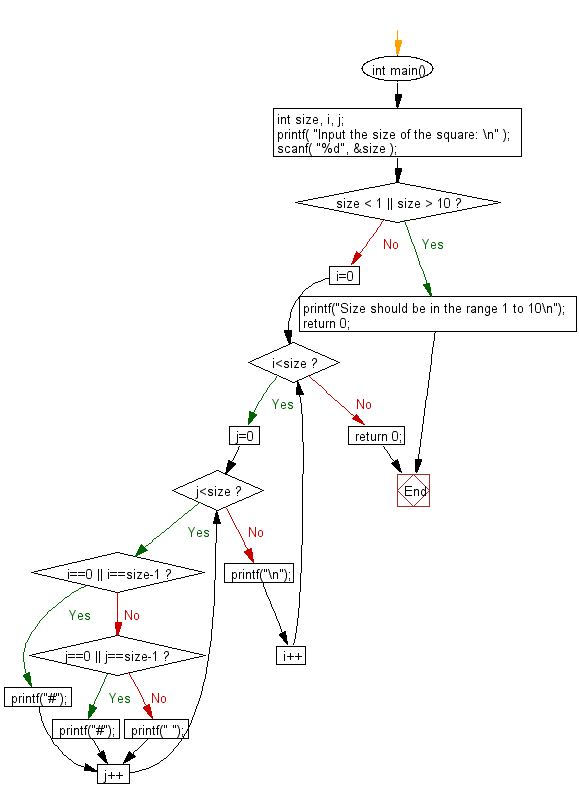﻿ C Program: Use a hash character to print a hollow square

# C Exercises: Reads the side of a square and prints a hollow square using hash (#) character

## C Basic Declarations and Expressions: Exercise-81 with Solution

Write a C program that reads the side (side sizes between 1 and 10 ) of a square and prints a hollow square using the hash (#) character.

Sample Input: 10

Sample Solution:

C Code:

``````#include <stdio.h>

int main()
{
// Declare variables
int size, i, j;

// Prompt user for input
printf("Input the size of the square: ");

// Read the size from user
scanf("%d", &size);

// Check if size is within valid range
if (size < 1 || size > 10) {
printf("Size should be in the range 1 to 10\n");
return 0; // Exit program if size is invalid
}

// Loop for rows
for (i = 0; i< size; i++)
{
// Loop for columns
for (j = 0; j < size; j++)
{
// Check if it's a border or inside space
if (i == 0 || i == size - 1)
printf("#"); // Print border
else if (j == 0 || j == size - 1)
printf("#"); // Print border
else
printf(" "); // Print space for inside
}
printf("\n"); // Move to the next line after each row is printed
}

return 0; // Indicate successful execution of the program
}
``````

Sample Output:

```Input the size of the square:
##########
#        #
#        #
#        #
#        #
#        #
#        #
#        #
#        #
##########
```

Pictorial Presentation:Flowchart:C programming Code Editor:

What is the difficulty level of this exercise?

Test your Programming skills with w3resource's quiz.

﻿